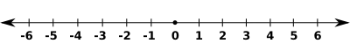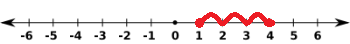How to Use a Number Line

Instructor: Cori Hodges

Cori has degrees in Elementary Education and Early Childhood Education and has taught lower elementary.

In this lesson you'll not only discover what a number line is, but you'll also learn how to use a number line to solve many math problems. Read on to learn more about number lines in this math lesson.

What Is a Number Line?

Did you know that a number line never ends? It also has no beginning. Doesn't that sound crazy?

A number line is a wonderful tool to help you with math. It's a straight line with numbers that increase or decrease, depending on which direction you're counting.

If you notice in the picture below, there are arrows at either end of the number line. That is because the numbers keep going. As far as you can count and even further. To the right, the numbers are growing, or increasing. To the left, the numbers are getting smaller, or decreasing. Once you go to left of the 0, the number line will include negative numbers, as shown below.Why Do We Need Number Lines?

Why do we need to use a number line? We have calculators and computers. Can't we just use those? Not always. Perhaps the batteries in your calculator are dead -- you can draw a number line! Maybe you're asked to show your work on a math problem -- you can draw a number line!

What Can Number Lines Do?

You can use a number line to add numbers, subtract numbers, compare numbers and count.

Whichever of these math problems you have, start by drawing a number line and writing the numbers in their correct order. The smaller the number, the closer it is to the start, or left side, of the line. The larger numbers are at the end, or right side, of the number line. If you are subtracting, you will be moving to the left of the line, and if you are adding, you will be moving to the right of the line.

Let's look at an example of a simple math problem. Let's say you want to figure out 1 + 3 using a number line. As shown in the example below, we'll start where the 1 is, and because we're adding, we'll count to the right three spaces. That tells us that 1 + 3 = 4.You can even use a number line for subtraction problems! For instance, using the image above, you could figure out that 4 - 3 = 1 by starting at the 4 and moving to the left, or subtracting, three spaces.

To unlock this lesson you must be a Study.com Member.

Register to view this lesson

Are you a student or a teacher?

See for yourself why 30 million people use Study.com

Become a Study.com member and start learning now.
Back
What teachers are saying about Study.com

Earning College Credit

Did you know… We have over 200 college courses that prepare you to earn credit by exam that is accepted by over 1,500 colleges and universities. You can test out of the first two years of college and save thousands off your degree. Anyone can earn credit-by-exam regardless of age or education level.